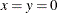### Model Update

The PROC OPTMODEL modeling language provides several means of modifying a model after it is first specified. You can change the parameter values of the model. You can add new model components. The FIX and UNFIX statements can fix variables to specified values or rescind previously fixed values. The DROP and RESTORE statements can deactivate and reactivate constraints. See also the section Multiple Subproblems for information on how to maintain multiple models.

To illustrate how these statements work, reconsider the following example from the section Constraints:

```proc optmodel;
var x, y;
min r = x**2 + y**2;
con c: x+y >= 1;
solve;
print x y;
```

As described previously, the solver finds the optimal point `x` = `y` = 0.5 with `r` = 0.5. You can see the effect of the constraint `c` on the solution by temporarily removing it. You can add the following statements:

```   drop c;
solve;
print x y;
```

This change produces the output in Figure 5.58.

Figure 5.58: Solution with Dropped Constraint

Problem Summary
Objective Sense Minimization
Objective Function r
Objective Type Quadratic

Number of Variables 2
Bounded Above 0
Bounded Below 0
Bounded Below and Above 0
Free 2
Fixed 0

Number of Constraints 0

Constraint Coefficients 0

Performance Information
Execution Mode Single-Machine
Number of Threads 4

Solution Summary
Solver QP
Algorithm Interior Point
Objective Function r
Solution Status Optimal
Objective Value 0

Primal Infeasibility 0
Dual Infeasibility 0
Bound Infeasibility 0
Duality Gap 0
Complementarity 0

Iterations 0
Presolve Time 0.00
Solution Time 0.00

x y
0 0

The optimal point is, as expected.

You can restore the constraint `c` with the RESTORE statement, and you can also investigate the effect of forcing the value of variable `x` to 0.3. This requires the following statements:

```   restore c;
fix x=0.3;
solve;
print x y c.dual;
```

This produces the output in Figure 5.59.

Figure 5.59: Solution with Fixed Variable

Problem Summary
Objective Sense Minimization
Objective Function r
Objective Type Quadratic

Number of Variables 2
Bounded Above 0
Bounded Below 0
Bounded Below and Above 0
Free 1
Fixed 1

Number of Constraints 1
Linear LE (<=) 0
Linear EQ (=) 0
Linear GE (>=) 1
Linear Range 0

Constraint Coefficients 0

Performance Information
Execution Mode Single-Machine
Number of Threads 4

Solution Summary
Solver QP
Algorithm Interior Point
Objective Function r
Solution Status Optimal
Objective Value 0.58

Primal Infeasibility 0
Dual Infeasibility 0
Bound Infeasibility 0
Duality Gap 0
Complementarity 0

Iterations 0
Presolve Time 0.00
Solution Time 0.01

x y c.DUAL
0.3 0.7 1.4

The variable `x` still has the value that was defined in the FIX statement. The objective value has increased by 0.08 from its constrained optimum 0.5 (see Figure 5.50). The constraint `c` is active, as confirmed by the positive dual value.

You can return to the original optimization problem by allowing the solver to vary variable `x` with the UNFIX statement, as follows:

```   unfix x;
solve;
print x y c.dual;
```

This produces the output in Figure 5.60. The model was returned to its original conditions.

Figure 5.60: Solution with Original Model

Problem Summary
Objective Sense Minimization
Objective Function r
Objective Type Quadratic

Number of Variables 2
Bounded Above 0
Bounded Below 0
Bounded Below and Above 0
Free 2
Fixed 0

Number of Constraints 1
Linear LE (<=) 0
Linear EQ (=) 0
Linear GE (>=) 1
Linear Range 0

Constraint Coefficients 2

Performance Information
Execution Mode Single-Machine
Number of Threads 4

Solution Summary
Solver QP
Algorithm Interior Point
Objective Function r
Solution Status Optimal
Objective Value 0.4999995397

Primal Infeasibility 2.5005E-7
Dual Infeasibility 2.3570226E-7
Bound Infeasibility 0
Duality Gap 1.9575231E-7
Complementarity 3.9804725E-8

Iterations 3
Presolve Time 0.00
Solution Time 0.01

x y c.DUAL
0.5 0.5 1